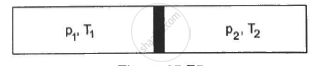Advertisement Remove all ads

# The figure shows an adiabatic cylindrical tube of volume V0 divided in two parts by a frictionless adiabatic separator - Physics

Answer in Brief

The figure shows an adiabatic cylindrical tube of volume V0 divided in two parts by a frictionless adiabatic separator. Initially, the separator is kept in the middle, an ideal gas at pressure p1 and temperature T1 is injected into the left part and another ideal gas at pressure p2 and temperature T2 is injected into the right part. Cp/Cv = γ is the same for both the gases. The separator is slid slowly and is released at a position where it can stay in equilibrium. Find (a) the volumes of the two parts (b) the heat given to the gas in the left part and (c) the final common pressure of the gases.Advertisement Remove all ads

#### Solution

For an adiabatic process, PVγ = Constant
So,  P1V1γ = P2V2γ   ...(i)
According to the problem,
V1 + V2 = V0  ...(ii)
Using the relation in eq (ii) in eq (i), we get
P1V1γ = P2(V0 − V1)γ

Or ("P"_1/"P"_2)^(1/gamma) = ("V"_0 -"V"_1)/"V"_1

"V"_1"P"_1^(1/gamma)  = "V"_0"P"_2^(1/gamma) - "V"_1"P"_2^(1/gamma)

"V"_1( "P"_1^(1/gamma) + "P"_2^(1/gamma)) = "V"_0"P"_2^(1/gamma)

"V"_1 = ("P"_2^(1-gamma) "V"_0)/("P"_1^(1/gamma) +"P"_2^(1/gamma))

Using equation (ii), we get

"V"_2 = ("P"_1^(1/gamma) "V"_0)/("P"_1 ^(1/gamma)+ "P"_2^(1/gamma))

(b) Since the whole process takes place in adiabatic surroundings, the separator is adiabatic.
Hence, heat given to the gas in the left part is 0.
(c) There will be a common pressure 'P' when equilibrium is reached. The slid will move until the pressure on the two sides becomes equal.
P1V1γ + P2V2γ = PV0γ

For equilibrium, "V"_1 = "V"_2 = "V"_0/2

Hence,

"P"_1("V"_0/2)^gamma + "P"_2("V"_0/2) ^gamma = "P"("V"_0)^gamma

Or "P" = (("P" _1^(1/gamma) + "P"_2^(1/gamma))/2)^gamma

Concept: Kinetic Theory of Gases and Radiation - Introduction of Kinetic Theory of an Ideal Gas
Is there an error in this question or solution?
Advertisement Remove all ads

#### APPEARS IN

HC Verma Class 11, Class 12 Concepts of Physics Vol. 2
Chapter 5 Specific Heat Capacities of Gases
Q 30 | Page 79
Advertisement Remove all ads
Advertisement Remove all ads
Share
Notifications

View all notifications

Forgot password?